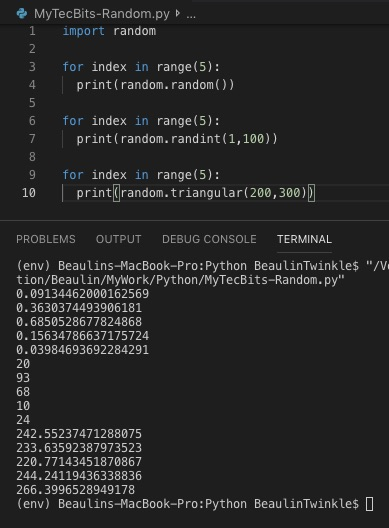# Generating random numbers in Python

In Python, there is a separate library module random for generating pseudo random numbers. This module has several options to generate random numbers. Here, we will see few of the random number generation methods which are useful during python programming.

## Random floating number

If you want floating-point random number between 0 and 1 then you can use the random() method under the random module. Here is an example

```>>> import random
>>> for index in range(5):
...   random.random()
...
0.09034640352214163
0.5567734810251059
0.6406356290715709
0.41040723367863097
0.485362051344318
>>>
```

## Random integer between two numbers

If you want integer random numbers between a range of numbers then use the randint() method. In randint() method, you can provide the maximum and the minimum integers as attributes. Here is an example to generate integer random numbers between 1 and 100.

```>>> import random
>>> for index in range(5):
...     random.randint(1,100)
...
71
36
91
76
41
>>>
```

## Random floating number between two numbers

Finally, to generate random floating-point numbers between a range of numbers, use the triangular() method. Here is an example. Here is an example to generate floating-point random numbers between 200 and 300.

```>>> import random
>>> for index in range(5):
...     random.triangular(200,300)
...
284.69384207547114
247.13877271206752
267.32853737609094
239.91254178747096
255.30147008066027
>>>
```

## Reference

### Related Articles & Tools

This site uses Akismet to reduce spam. Learn how your comment data is processed.